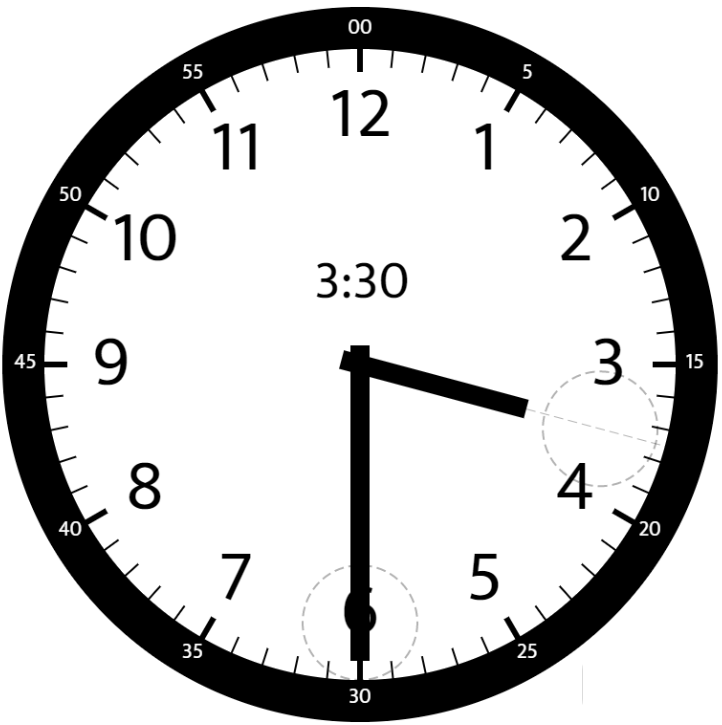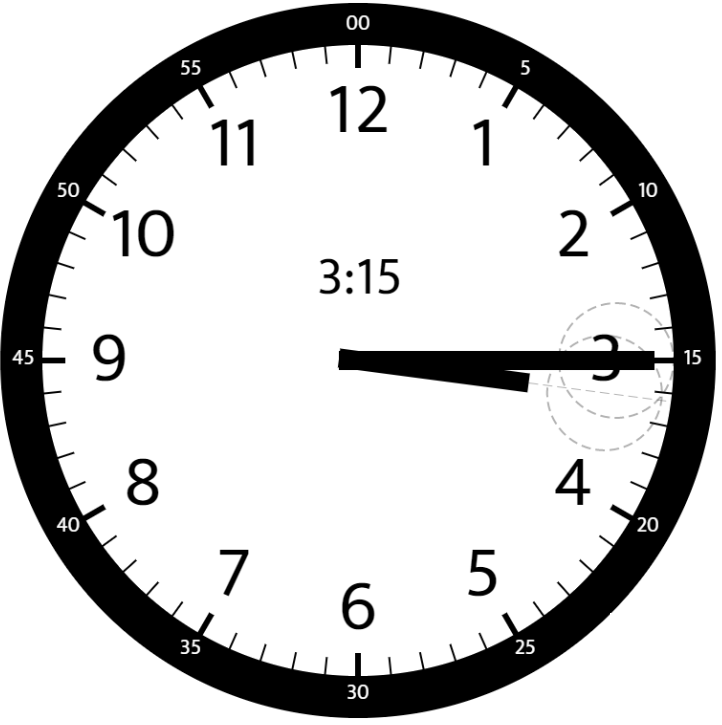## 1344. Angle Between Hands of a Clock

Given two numbers, `hour` and `minutes`. Return the smaller angle (in degrees) formed between the `hour` and the `minute` hand.

Example 1:```Input: hour = 12, minutes = 30
Output: 165
```

Example 2:```Input: hour = 3, minutes = 30
Output: 75
```

Example 3:```Input: hour = 3, minutes = 15
Output: 7.5
```

Example 4:

```Input: hour = 4, minutes = 50
Output: 155
```

Example 5:

```Input: hour = 12, minutes = 0
Output: 0
```

Constraints:

• `1 <= hour <= 12`
• `0 <= minutes <= 59`
• Answers within `10^-5` of the actual value will be accepted as correct.

## Rust Solution

``````struct Solution;

impl Solution {
fn angle_clock(hour: i32, minutes: i32) -> f64 {
let h = ((hour % 12) as f64 + (minutes as f64 / 60.0)) * 30.0;
let m = minutes as f64 * 6.0;
let a = (h - m).abs();
if a > 180.0 {
360.0 - a
} else {
a
}
}
}

#[test]
fn test() {
use assert_approx_eq::assert_approx_eq;
let hour = 12;
let minutes = 30;
let res = 165.0;
assert_approx_eq!(Solution::angle_clock(hour, minutes), res);
let hour = 3;
let minutes = 30;
let res = 75.0;
assert_approx_eq!(Solution::angle_clock(hour, minutes), res);
let hour = 3;
let minutes = 15;
let res = 7.5;
assert_approx_eq!(Solution::angle_clock(hour, minutes), res);
let hour = 4;
let minutes = 50;
let res = 155.0;
assert_approx_eq!(Solution::angle_clock(hour, minutes), res);
let hour = 12;
let minutes = 0;
let res = 0.0;
assert_approx_eq!(Solution::angle_clock(hour, minutes), res);
let hour = 1;
let minutes = 57;
let res = 76.5;
assert_approx_eq!(Solution::angle_clock(hour, minutes), res);
}
``````

Having problems with this solution? Click here to submit an issue on github.